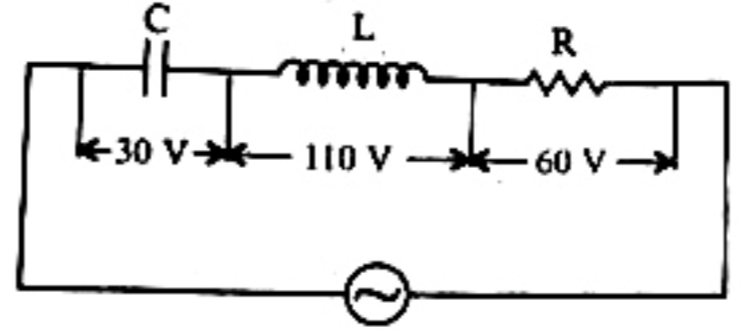# Q. In the given circuit the peak voltage across C, L and R are 30 V, 110 V and 60 V respectively. The rms value of the applied voltage isKCETKCET 2020

Solution:

## 1. The condition for a uniform spherical mass m of radius r to be a black hole is [G = gravitational constant and g = acceleration due to gravity]

AIIMS 2005 Gravitation

KCET 2020

## 3. The charge deposited on 4 $\mu$F capacitor in the circuit is

KCET 2009 Electrostatic Potential And Capacitance

KCET 2020

## 5. The potential to which a conductor is raised, depends on ........

KCET 2005 Electrostatic Potential And Capacitance

KCET 2020

KCET 2020

KCET 2020

KCET 2020

## 10. In the circuit shown, the currents $i_1$ and $i_2$ are ___________

KCET 2011 Current Electricity